• +91 9971497814
• info@interviewmaterial.com

# Chapter 1- Some Basic Concepts of Chemistry Interview Questions Answers

### Related Subjects

Question 1 :

Calculate the molecular mass of the following:-

(i) H2O (ii) CO2 (iii)CH4

(i) H2O:

The molecular mass of water, H2O

= (2 ×Atomic mass of hydrogen) + (1 × Atomic mass of oxygen)

=[2(1.0084) + 1(16.00 u)]

= 2.016 u +16.00 u

= 18.016

= 18.02 u

(ii) CO2:

The molecular mass of carbon dioxide, CO2

= (1 ×Atomic mass of carbon) + (2 × Atomic mass of oxygen)

= [1(12.011u) + 2 (16.00 u)]

= 12.011 u+ 32.00 u

= 44.01 u

(iii) CH4:

The molecular mass of methane, CH4

= (1 ×Atomic mass of carbon) + (4 × Atomic mass of hydrogen)

= [1(12.011u) + 4 (1.008 u)]

= 12.011 u+ 4.032 u

= 16.043 u

Question 2 :

Calculate the mass percent of differentelements present in sodium sulphate (Na2SO4).

The molecular formula of sodium sulphate is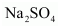.

Molar mass of= [(2 × 23.0) + (32.066) + 4 (16.00)]

= 142.066 g

Mass percent of an element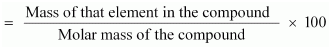Masspercent of sodium: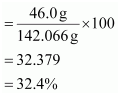Mass percent of sulphur: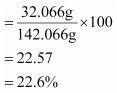Mass percent of oxygen: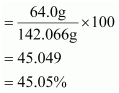Question 3 :

Determine the empirical formula of an oxide of iron whichhas 69.9% iron and 30.1% dioxygen by mass.

% of iron by mass = 69.9 % [Given]

% of oxygenby mass = 30.1 % [Given]

Relativemoles of iron in iron oxide: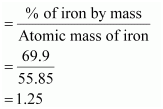Relative moles of oxygen in iron oxide: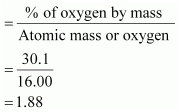Simplest molar ratio of iron to oxygen:

= 1.25:1.88

= 1: 1.5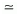2: 3

The empirical formula of the iron oxide is Fe2O3.

Question 4 :

Calculate the amount of carbon dioxide that could beproduced when

(i) 1 moleof carbon is burnt in air.

(ii) 1 moleof carbon is burnt in 16 g of dioxygen.

(iii) 2 moles of carbon are burnt in 16 g of dioxygen.

The balanced reaction of combustion of carbon can bewritten as: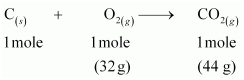(i) As per the balanced equation, 1 mole of carbon burns in1mole of dioxygen (air) to produce1 mole of carbon dioxide.

(ii) According to the question, only 16 g of dioxygen isavailable. Hence, it will react with 0.5 mole of carbon to give 22 g of carbondioxide. Hence, it is a limiting reactant.

(iii) According to the question, only 16 g of dioxygen isavailable. It is a limiting reactant. Thus, 16 g of dioxygen can combine withonly 0.5 mole of carbon to give 22 g of carbon dioxide.

Question 5 :

Calculate the mass of sodium acetate (CH3COONa) required to make 500 mL of 0.375 molaraqueous solution. Molar mass of sodium acetate is 82.0245 g mol–1

0.375 M aqueous solution of sodium acetate

≡ 1000 mLof solution containing 0.375 moles of sodium acetate

Number of moles of sodium acetate in 500 mL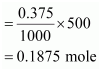Molar mass of sodium acetate = 82.0245 g mole–1 (Given)

Requiredmass of sodium acetate = (82.0245 g mol–1)(0.1875 mole)

= 15.38 g

Question 6 :

Calculate the concentration of nitric acidin moles per litre in a sample which has a density, 1.41 g mL–1 and the mass per cent of nitric acid in itbeing 69%.

Mass percent of nitric acid in the sample = 69 % [Given]

Thus, 100 gof nitric acid contains 69 g of nitric acid by mass.

Molar mass of nitric acid (HNO3)

= {1 + 14 + 3(16)} g mol–1

= 1 + 14 +48

= 63 g mol–1

Number ofmoles in 69 g of HNO3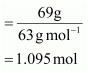Volume of 100g of nitric acid solution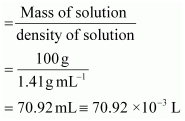Concentration of nitric acid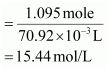Concentrationof nitric acid = 15.44 mol/L

Question 7 :

How much copper can be obtained from 100 gof copper sulphate (CuSO4)?

1 mole of CuSO4 contains1 mole of copper.

Molar mass of CuSO4 =(63.5) + (32.00) + 4(16.00)

= 63.5 +32.00 + 64.00

= 159.5 g

159.5 g of CuSO4 contains63.5 g of copper.

100 g of CuSO4 will contain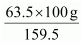of copper.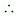Amountof copper that can be obtained from 100 g CuSO4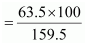= 39.81 g

Question 8 :

Determine the molecular formula of an oxideof iron in which the mass per cent of iron and oxygen are 69.9 and 30.1respectively. Given that the molar mass of the oxide is 159.69 g mol–1.

Mass percent of iron (Fe) = 69.9% (Given)

Masspercent of oxygen (O) = 30.1% (Given)

Number of moles of iron present in the oxide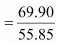= 1.25

Number of moles of oxygenpresent in the oxide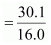= 1.88

Ratio ofiron to oxygen in the oxide,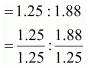= 1 : 1.5

= 2 : 3Theempirical formula of the oxide is Fe2O3.

Empirical formula mass of Fe2O3 = [2(55.85) + 3(16.00)] g

Molar mass of Fe2O3 = 159.69 g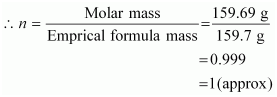Molecular formula of a compound is obtainedby multiplying the empirical formula with n.

Thus, the empirical formula of the givenoxide is Fe2O3 and n is 1.

Hence, the molecular formula of the oxide isFe2O3.

Question 9 :

Calculate the atomic mass (average) of chlorine using thefollowing data:

 % Natural Abundance Molar Mass 35Cl 75.77 34.9689 37Cl 24.23 36.9659

The average atomic mass of chlorine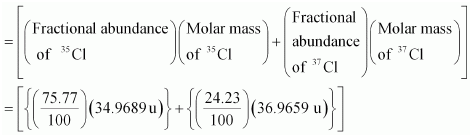= 26.4959 + 8.9568

= 35.4527 uThe averageatomic mass of chlorine = 35.4527 u

Question 10 :

In three moles of ethane (C2H6),calculate the following:

(i) Number of moles of carbon atoms.

(ii) Number of moles of hydrogen atoms.

(iii) Number of molecules of ethane.

(i) 1 mole of C2H6 contains 2 molesof carbon atoms.Number ofmoles of carbon atoms in 3 moles of C2H6

= 2 × 3 = 6

(ii) 1 mole of C2Hcontains 6 molesof hydrogen atoms.Number ofmoles of carbon atoms in 3 moles of C2H6

= 3 × 6 = 18

(iii) 1 mole of C2Hcontains 6.023 ×1023 molecules of ethane.Number ofmolecules in 3 moles of C2H6

= 3 × 6.023 × 1023 = 18.069× 1023

Todays Deals### Chapter 1- Some Basic Concepts of Chemistry Contributorskrishan

Name:
Email:

# Latest News# 9000 interview questions in different categories